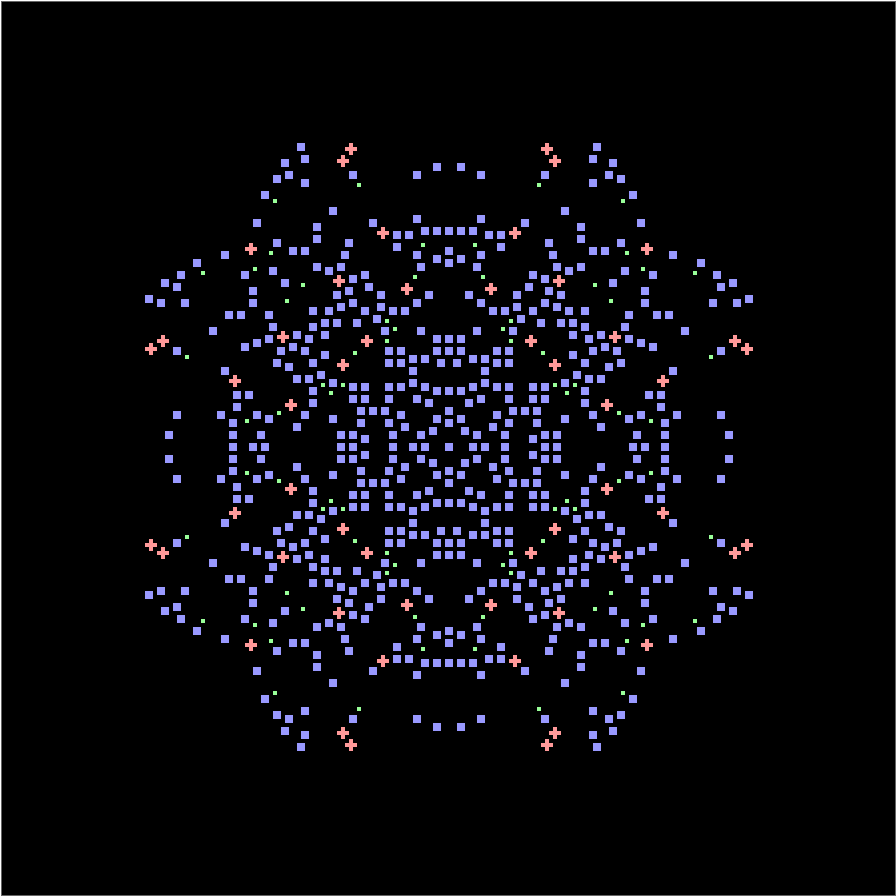Function Repository Resource:

# SubmatrixReplace

Efficiently replace rectangular submatrices of an input data matrix

 ResourceFunction["SubmatrixReplace"][data,rules] applies rules to all relevant rectangular submatrices of the data matrix, by default taking the first sorted value when replacements collide on an element.

## Details

Each rule in rules must map between submatrices of fixed rectangular dimensions m×n, but m and n may vary across rules.
The replacement procedure groups rules by submatrix dimension, and applies them to individual submatrices using ReplaceAll.
When application of rules over all submatrices leads to two or more plausible image values for one particular matrix element, a collision is said to occur.
Collisions occur in two different cases:
Applying only one replacement rule may cause a collision within a region where two submatrices overlap.
Applying many distinct replacement rules may cause a collision within a region where two submatrices overlap.
Collisions do not occur in only one submatrix, because the underlying mechanism involves ReplaceAll.
ResourceFunction["SubmatrixReplace"] takes the following options:
 "Cyclic" {True,True} determines cyclic or terminal boundary conditions for row and columns CollisionFunction First[#2]& determines how to resolve collisions Method "Structured" specifies how to handle submatrices
The CollisionFunction acts locally on elements by returning an output value from three sequential inputs:
 #1 a single initial value #2 a list containing the stack of candidate replacement values #3 a list containing row and column indices
Choosing Method "Structured" uses the resource function BlockSubmatrices to build an optimized data structure.
Choosing Method "Simple" uses raw output from Partition.

## Examples

### Basic Examples (3)

Replace the central diagonal of an identity matrix:

 In:=Out=Use a functional notation to trim the output of DiamondMatrix:

 In:=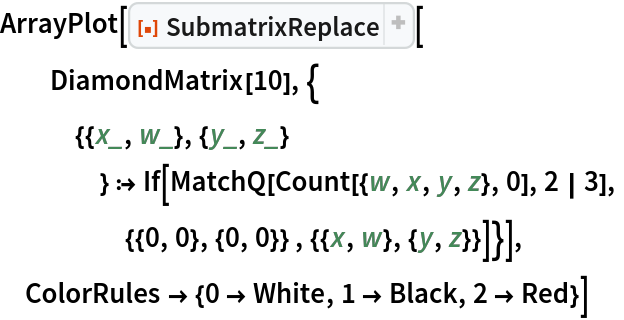Out=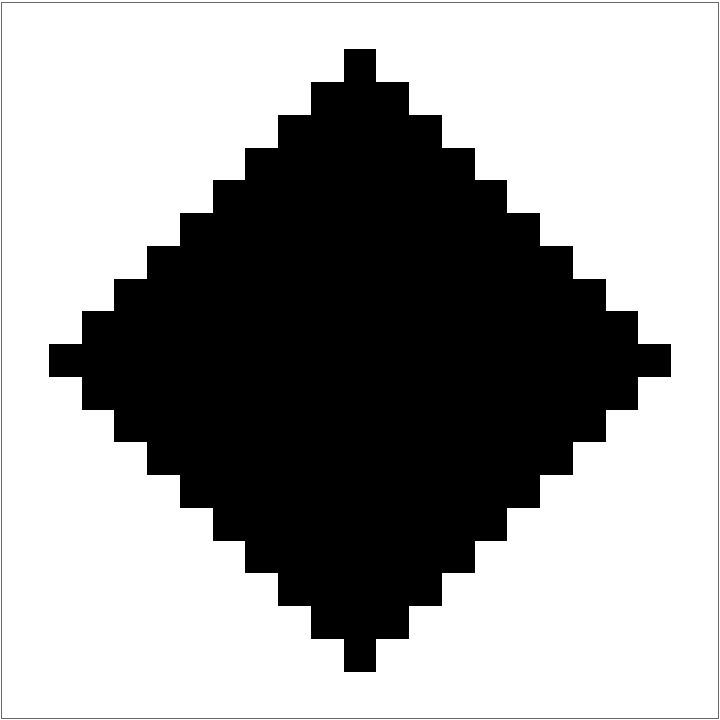Notice collision stacking when highlighting pattern fragments within a HadamardMatrix output:

 In:=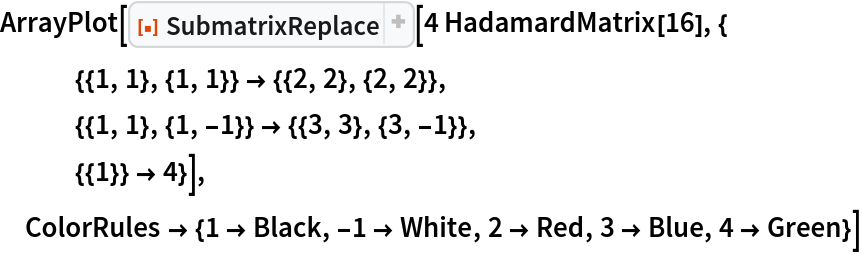Out=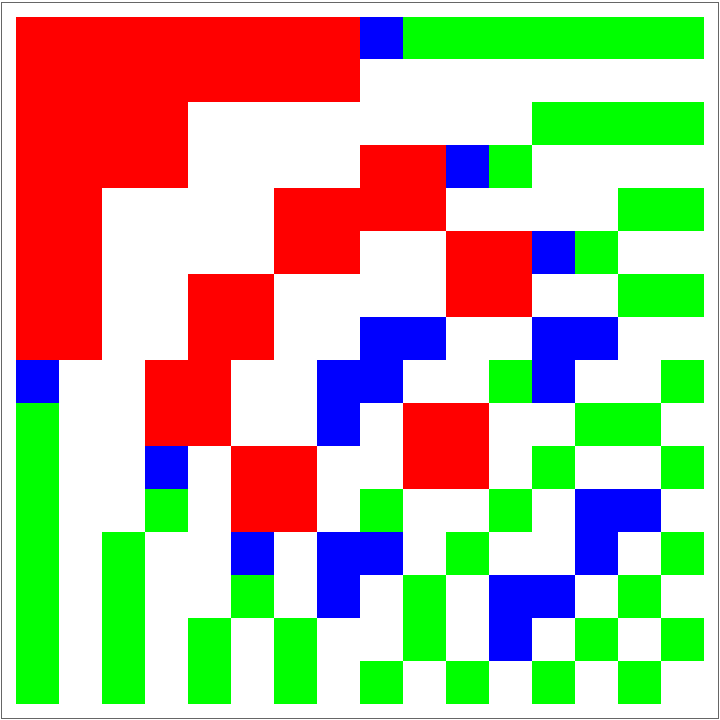Change resolution of collisions by setting the CollisionFunction option:

 In:=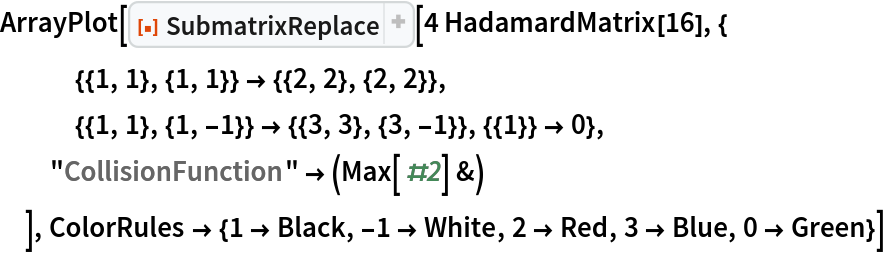Out=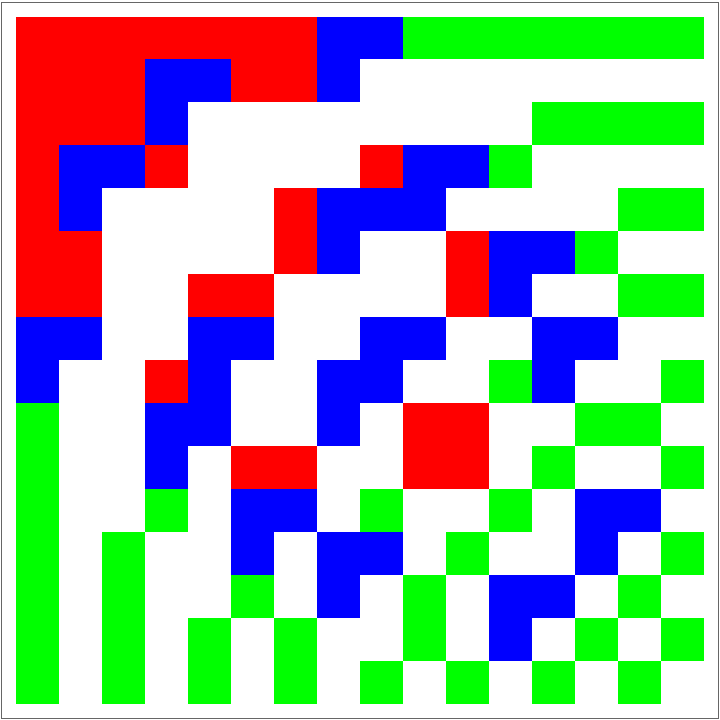### Scope (3)

Test occurrence of certain blocks in a pseudorandom matrix pattern:

 In:=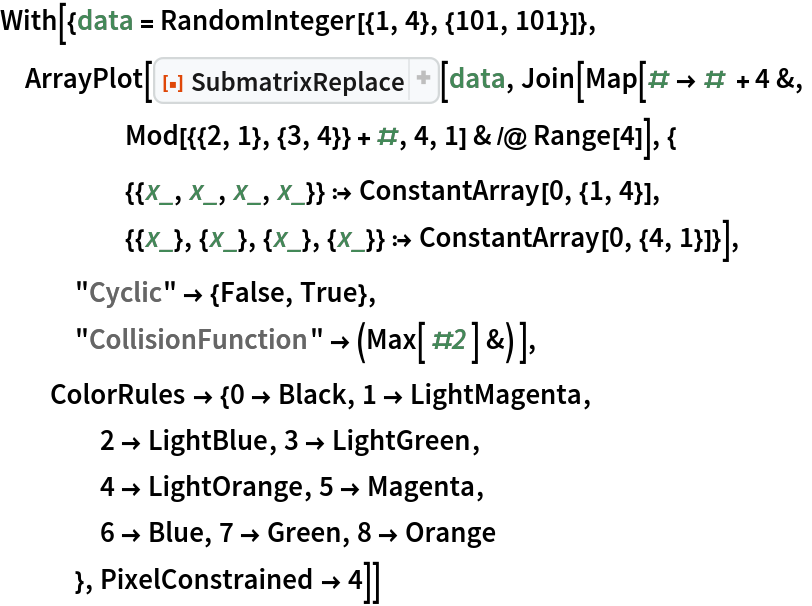Out=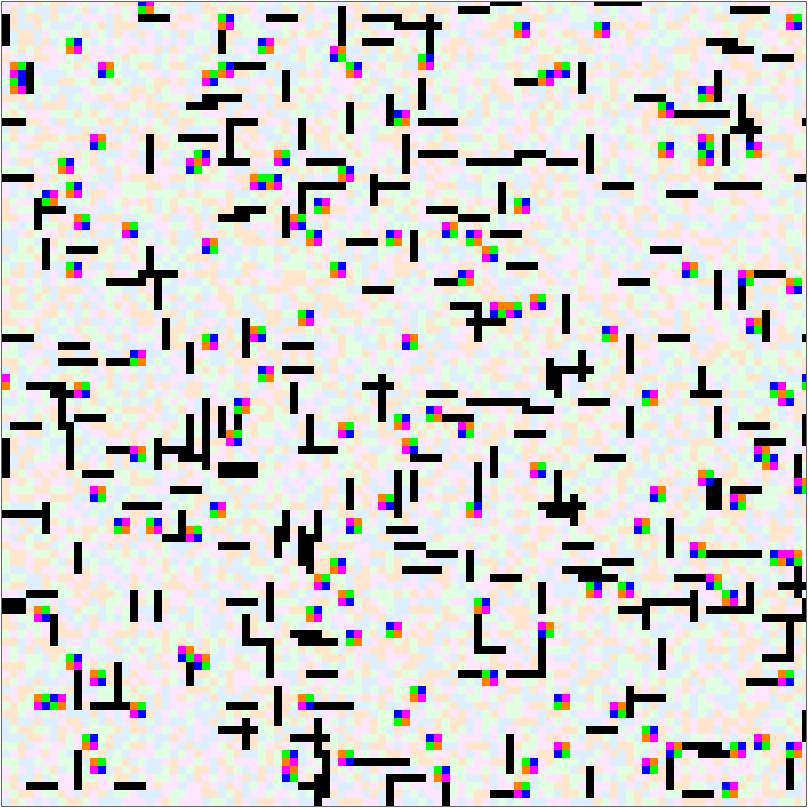Restrict search range to a central patch:

 In:=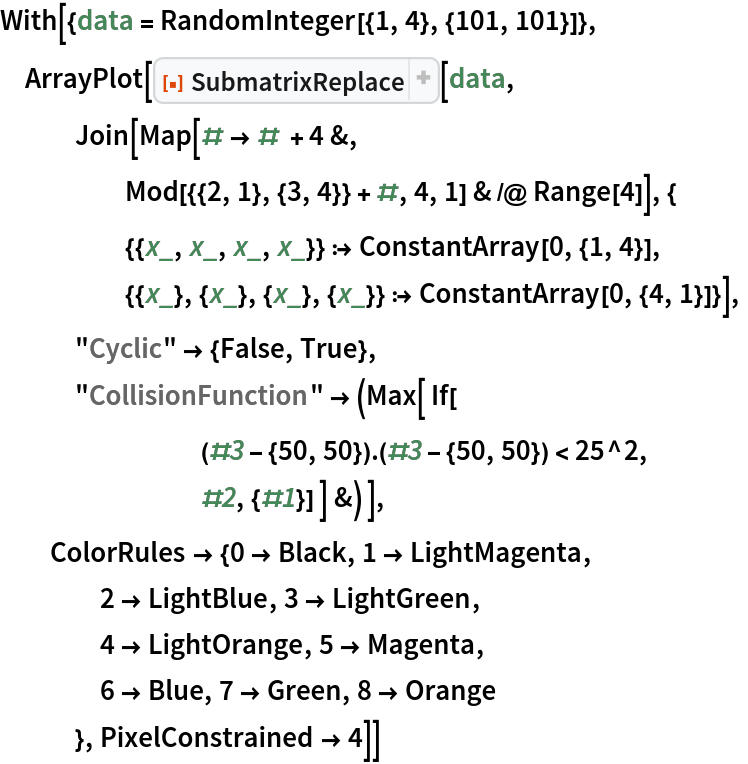Out=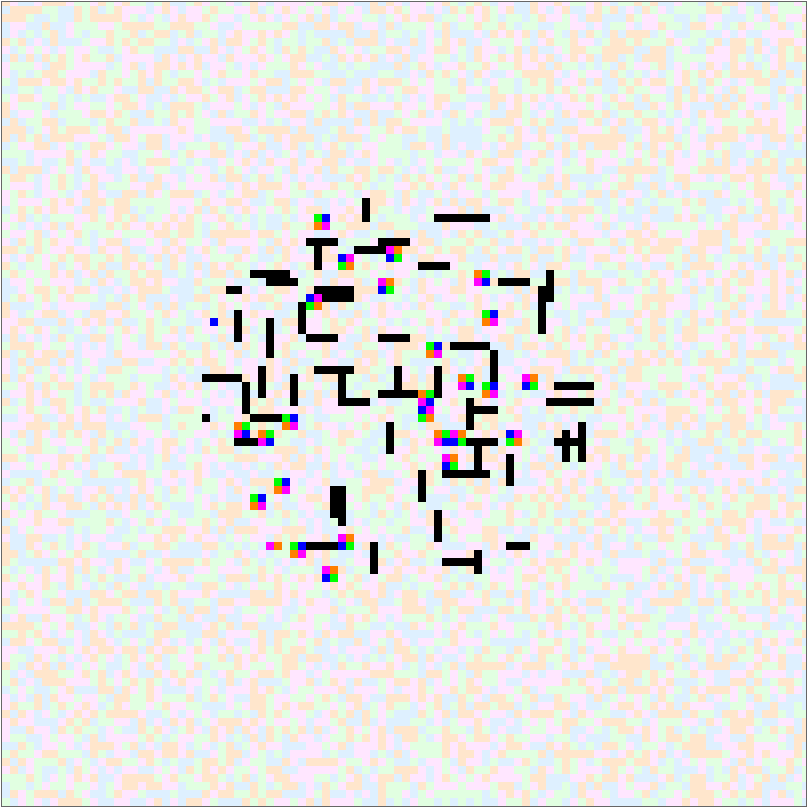Timing test Method Simple against the task of highlighting Rule 110's periodic background:

 In:=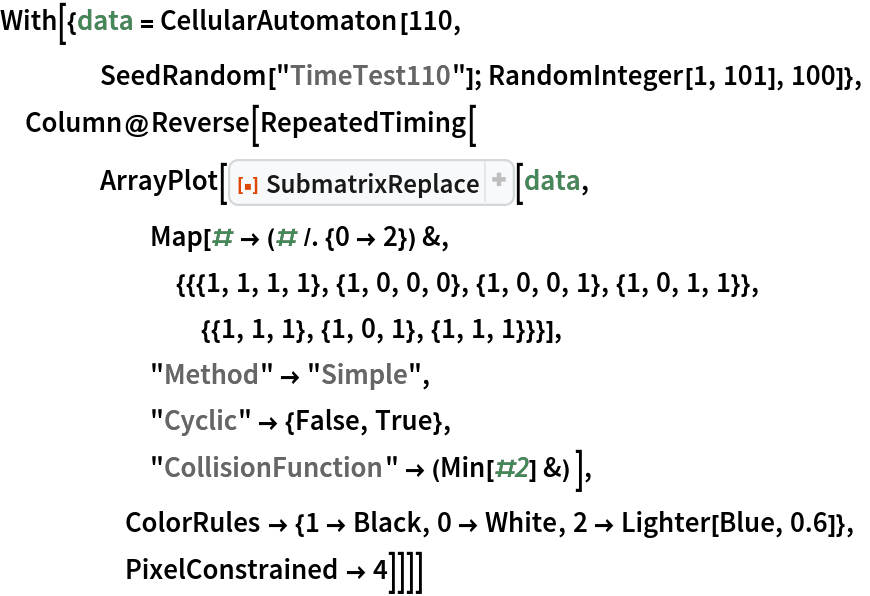Out=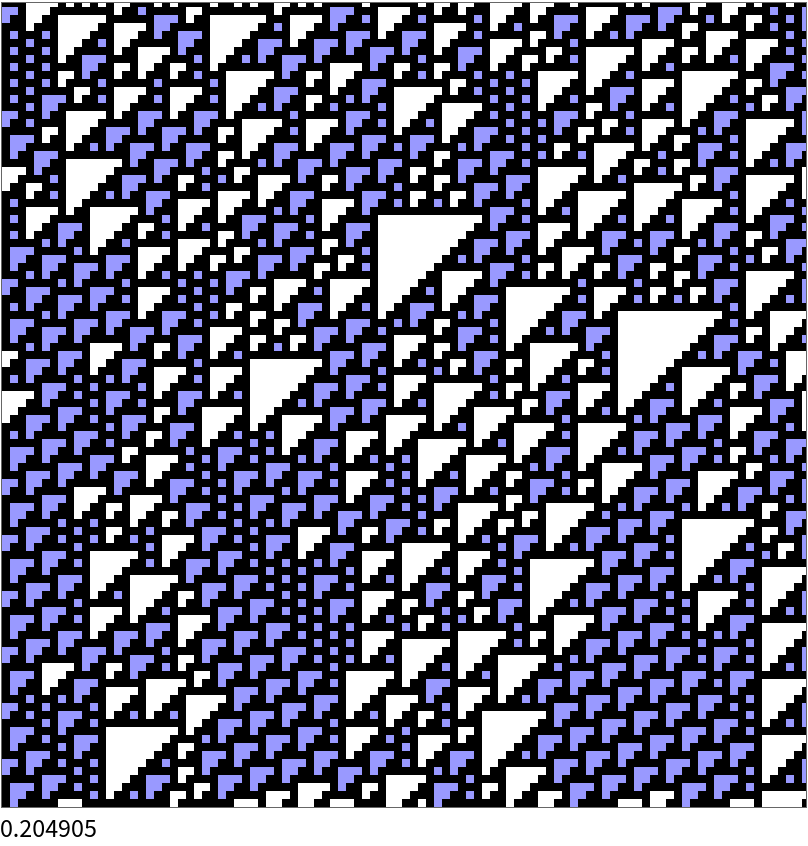Repeat timing test with default Method Structured:

 In:=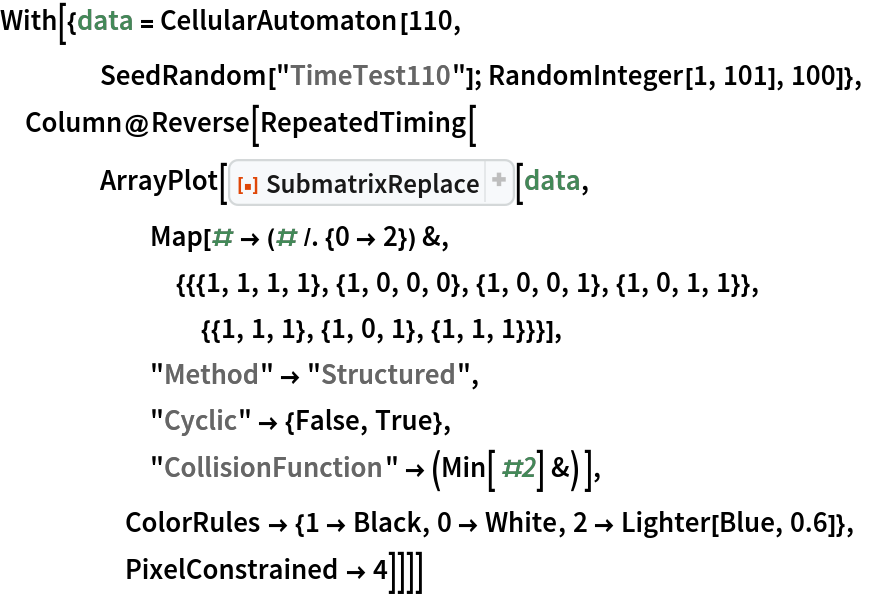Out=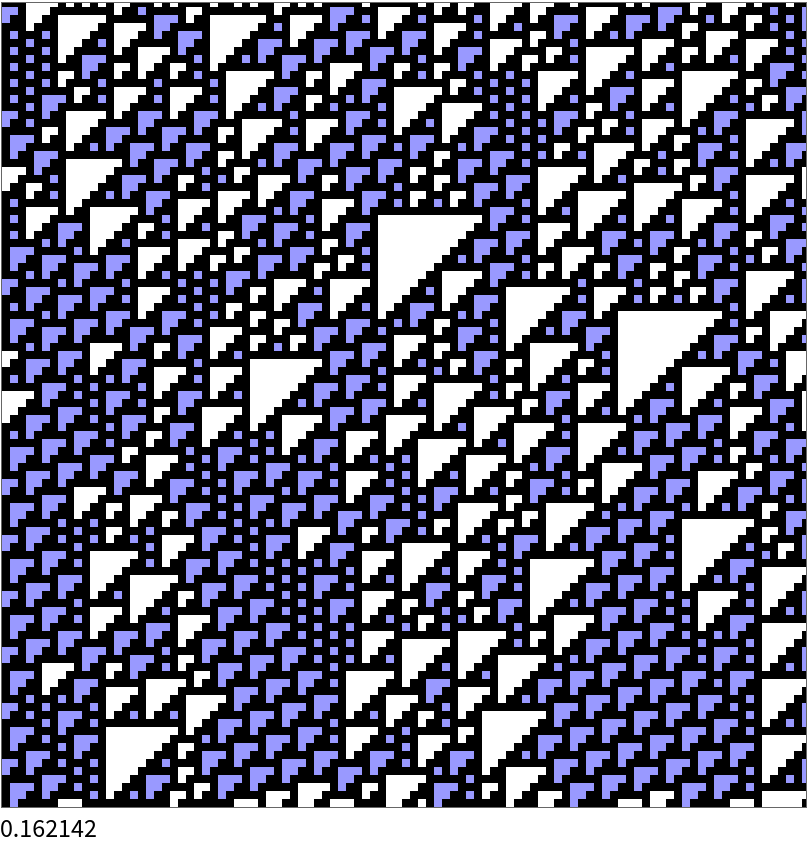Compare complex-valued collision alternatives by inspecting elements:

 In:=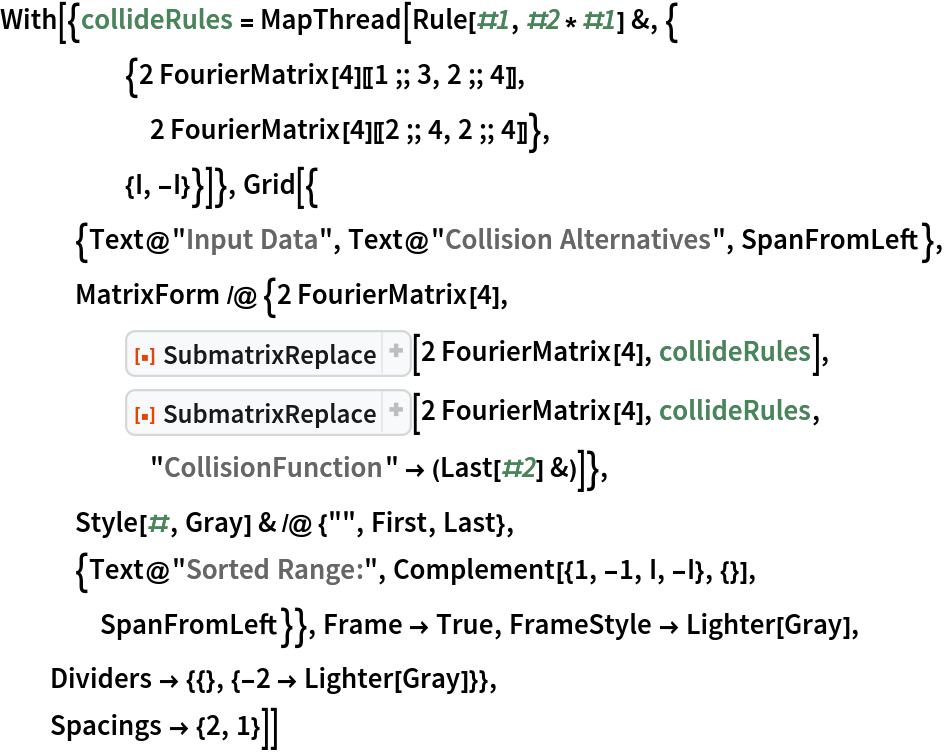Out=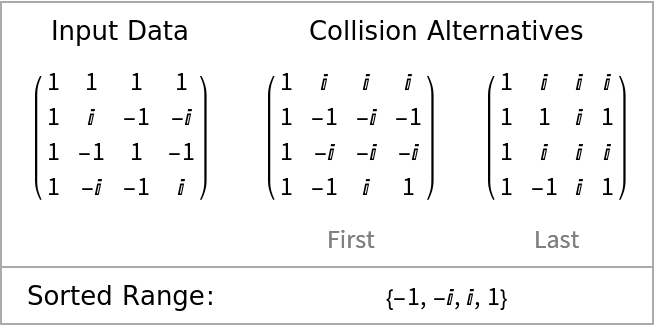Even using only one rule, a collision can still occur:

 In:=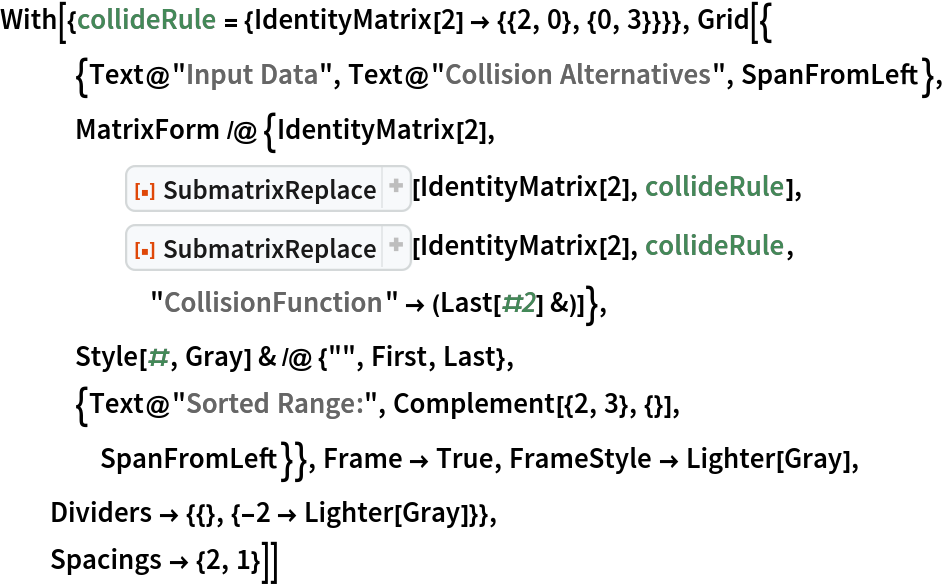Out=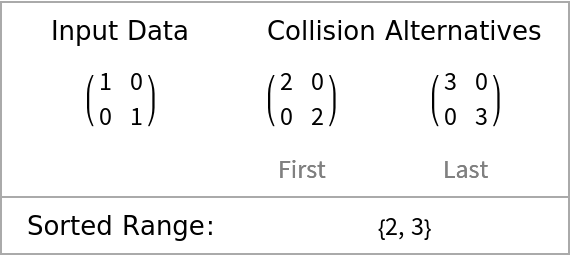### Neat Examples (1)

Highlight persistent structures in a 2D Cellular Automaton output:

 In:=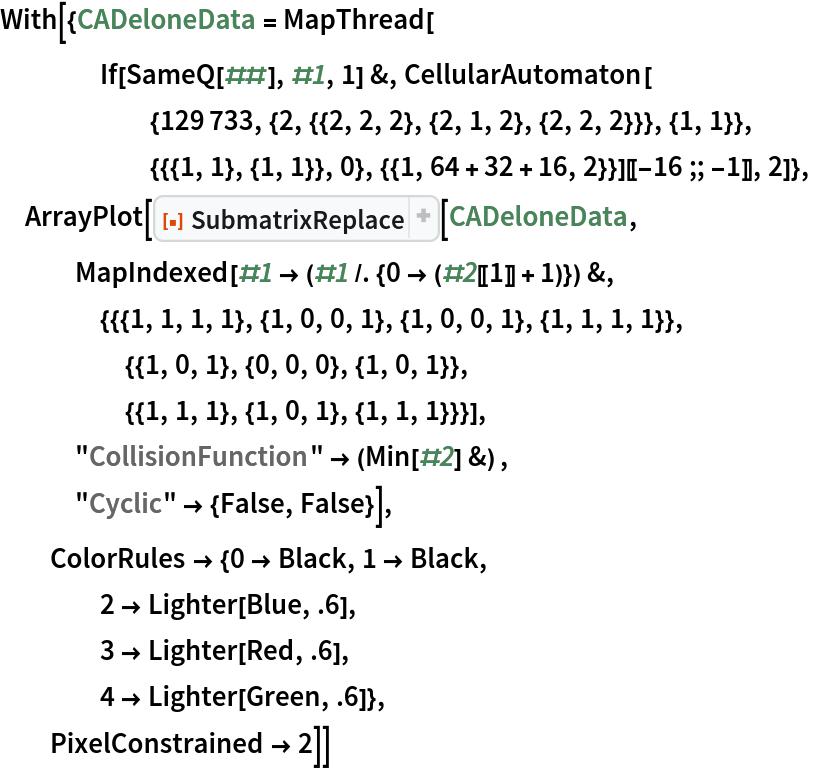Out=